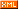# Nowpaper 十五英寸的世界

Rich Games Developer

 博客园  :: 首页  :: 新随笔  :: 联系 :: 订阅:: 管理

private onclick_level(e:egret.TouchEvent){
var icon = <LevelIcon>e.currentTarget;
if(this.sel_level != icon.Level){
this.img_arrow.x = icon.x;
this.img_arrow.y = icon.y;
this.sel_level = icon.Level;
}else{
//进入并开始游戏
SceneGame.Shared().InitLevel(icon.Level);
this.parent.removeChild(this);
}
}

class SceneGame extends eui.Component {
//单例
private static shared: SceneGame;
public static Shared() {
if(SceneGame.shared == null) {
SceneGame.shared = new SceneGame();
}
return SceneGame.shared;
}
public constructor() {
super();
this.skinName = "src/Game/SceneGameSkin.exml";

}
//对象变量
private group_words: eui.Group;
private img_question: eui.Image;
private btn_back: eui.Group;
private levelIndex:number;
//初始化关卡
public InitLevel(level:number){
this.levelIndex = level;
var leveldata = LevelDataManager.Shared().GetLevel(level);
}
private onclick_back(){
this.parent.removeChild(this);
}
}

//初始化关卡
public InitLevel(level:number){
this.levelIndex = level;
var leveldata = LevelDataManager.Shared().GetLevel(level);
//将字段接起来
var words = leveldata.answer + leveldata.word;
//随机一个其它题目的字段混合进本题目
while(words.length==10){
var i = Math.floor(Math.random() * 400);
if(i!=level){
var temp = LevelDataManager.Shared().GetLevel(i);
}
}
//对字段重排
var wordlist:string[]=[];
for(var i =0 ;i<words.length;i++){
wordlist.push(words.charAt(i));
}
wordlist = this.randomlist(wordlist);
//赋值
for(var i = 0;i<this.group_words.numChildren;i++){
var wordrect = <Word>this.group_words.getChildAt(i);
wordrect.setWordText(wordlist[i]);
wordrect.visible = true;
}
//重置一些状态
}
//显示图像
this.img_question.source = "resource/assets/"+leveldata.img;
}
//将一个数列随机
private randomlist(arr: any[]): any[] {
var array = [];
while(arr.length > 0) {
var i = Math.floor(Math.random() * arr.length);
array.push(arr[i]);
arr.splice(i,1);
}
return array;
}

//当一个问题字被选择添加到回答的时，设置不可见，并保存到本对象中以后使用
public SetSelectWord(word:Word){
if(word != null){
this.setWordText(word.getWordText());
this.SelectWord = word;
word.visible = false;
}
else{
this.setWordText("");
this.SelectWord = null;
}
}

protected onclick_tap(){
SceneGame.Shared().onclick_word(this);
}

//当字点击的时候，由word类抛出
public onclick_word(word: Word){
//找到一个合适的位置添加进答案内容

break;
}
}
//当有一个合适的位置的时候就会将字填充，并判断是否胜利
if(sel != null){
sel.SetSelectWord(word);
//判断是否胜利
var check_str:string = "";
for(var i = 0;i < this.group_answer.numChildren;i++) {
}
//胜利
console.log("win");
}
}
}

protected startCreateScene(): void {
}